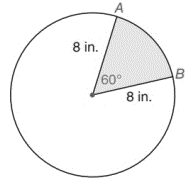Chapter 8.4, Problem 9E### Elementary Geometry for College St...

6th Edition
Daniel C. Alexander + 1 other
ISBN: 9781285195698

#### Solutions

Chapter
Section### Elementary Geometry for College St...

6th Edition
Daniel C. Alexander + 1 other
ISBN: 9781285195698
Textbook Problem
1 views

# Find exactly l A B ⌢ , where A B ⌢ refers to the minor arc of the circle.To determine

To Find:

The length of AB, where AB refers to the minor arc of the circle.

Explanation

To find the length of the arc, first find the circumference of the circle.

The circumference of the circle, d=2r.

It is given that the radius of the circle is 8 in.

Therefore, C=23.148=50.24.

Therefore, the circumference of the circle is 50.24 in.

Next, the degree of the arc AB is 60°.

The degree of the entire circle is 360°.

The ratio of the degree of the arc (m) to the degree of the entire circle (360) is the same as the ratio of the length of the arc to the circumference of the circle

### Still sussing out bartleby?

Check out a sample textbook solution.

See a sample solution

#### The Solution to Your Study Problems

Bartleby provides explanations to thousands of textbook problems written by our experts, many with advanced degrees!

Get Started

#### Find more solutions based on key concepts